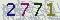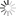﻿ 数学学院珠峰讲坛：Asymptotics of The Density of Parabolic Anderson Random Fields -山东大学新闻网### 数学学院珠峰讲坛：Asymptotics of The Density of Parabolic Anderson Random Fields

Asymptotics of The Density of Parabolic Anderson Random Fields

Parabolic Anderson model is a very simple stochastic heat equation with multiplicative Gaussian noise. The solution $u(t,x)$ of this equation can be represented by the Wiener-It\^o chaos expansion. It is related to the Anderson localization and is also related to the so-called KPZ equation describing the physical growth phenomena. We investigate the shape of the density ρ(t,x; y) of the solution u(t,x) to the stochastic partial differential equation $\frac{\partial}{\partial t} u(t,x) = (1/2) \Delta u(t,x)+u \diamond \dot W (t,x)$, where $\dot W (t,x)$ is a general Gaussian noise and $\diamond$ denotes the Wick product. We mainly concern with the asymptotic behavior of $\rho(t,x; y)$, the density of the random variable $u(t,x)$, when $y\to\infty$ or when $t\to 0+$. Both upper and lower bounds are obtained and these two bounds match each other modulo some multiplicative constants. If the initial condition is positive, then $\rho(t,x; y)$ is supported on the positive half-line $y\in [0, \infty)$ and in this case we show that $\rho(t,x; 0+)=0$ and obtain an upper bound for $\rho(t,x; y)$ when $y\to 0+$. Our tool is Malliavin calculus and I will also present a very brief and heuristic introduction.

This is joint work with Khoa Le.

8月9日（周二）10:00--11:00

【作者：张志越    来自：数学学院    编辑：新闻网工作室    责任编辑：王亦婷 赵方方 　】

 验证码看不清楚,换张图片 共0条评论    共1页   当前第1页 拖动光标可翻页查看更多评论

### 最新发布### 新闻排行• 山大日记
• 山大人物
• 视点微信
• 互动话题
• 视点图志
• 精彩视频